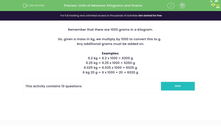# Units of Measure: Kilograms and Grams

In this worksheet, students state how many grams there are in masses given in kilograms and grams.Key stage:  KS 2

Curriculum topic:   Measurement

Curriculum subtopic:   Convert Between Metric Measurements

Difficulty level:#### Worksheet Overview

Remember that there are 1000 grams in a kilogram.

So, given a mass in kg, we multiply by 1000 to convert this to g.

Examples:

6.2 kg = 6.2 x 1000 = 6200 g

6.25 kg = 6.25 x 1000 = 6250 g

6.025 kg = 6.025 x 1000 = 6025 g

6 kg 20 g = 6 x 1000 + 20 = 6020 g

### What is EdPlace?

We're your National Curriculum aligned online education content provider helping each child succeed in English, maths and science from year 1 to GCSE. With an EdPlace account you’ll be able to track and measure progress, helping each child achieve their best. We build confidence and attainment by personalising each child’s learning at a level that suits them.

Get started